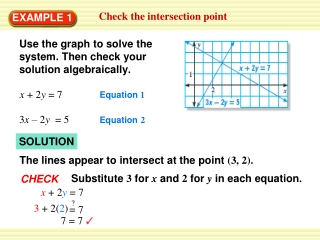DownloadDownload Presentation7 = 7

# 7 = 7

Télécharger la présentation## 7 = 7

- - - - - - - - - - - - - - - - - - - - - - - - - - - E N D - - - - - - - - - - - - - - - - - - - - - - - - - - -
##### Presentation Transcript

1. 7 = 7 ? = 3+ 2(2) 7 Check the intersection point EXAMPLE 1 Use the graph to solve the system. Then check your solution algebraically. x + 2y = 7 Equation 1 Equation2 3x – 2y = 5 SOLUTION The lines appear to intersect at the point (3, 2). Substitute3forxand2foryin each equation. CHECK x+ 2y= 7

2. 5 3(3) – 2(2) 5 = 5 ANSWER Because the ordered pair (3, 2) is a solution of each equation, it is a solution of the system. ? = Check the intersection point EXAMPLE 1 3x– 2y= 5

3. Use the graph-and-check method EXAMPLE 2 Solve the linear system: –x + y = –7 Equation 1 Equation2 x + 4y = –8 SOLUTION STEP1 Graph both equations.

4. –8 = –8 –(4) + (–3) –7 ? ? 4+ 4(–3) –8 = = –7= –7 Use the graph-and-check method EXAMPLE 2 STEP2 Estimate the point of intersection. The two lines appear to intersect at (4, – 3). STEP3 Check whether (4, –3) is a solution by substituting 4 for xand –3 for yin each of the original equations. Equation1 Equation2 –x+y=–7 x+4y=–8

5. ANSWER Because (4, –3) is a solution of each equation, it is a solution of the linear system. Use the graph-and-check method EXAMPLE 2

6. 1. –5x + y = 0 5x + y = 10 ANSWER (1, 5) Use the graph-and-check method EXAMPLE 2 for Examples 1 and 2 GUIDED PRACTICE Solve the linear system by graphing. Check your solution.

7. 2. –x + 2y = 3 2x + y = 4 ANSWER (1, 2) Use the graph-and-check method EXAMPLE 2 for Examples 1 and 2 GUIDED PRACTICE Solve the linear system by graphing. Check your solution.

8. x – y = 5 3. 3x + y = 3 ANSWER (2, 3) Use the graph-and-check method EXAMPLE 2 for Examples 1 and 2 GUIDED PRACTICE Solve the linear system by graphing. Check your solution.